RS Aggarwal Class 8 Solutions Chapter 25 - Graphs

RS Aggarwal Class 8 Chapter 25 - Graphs Solutions Free PDF

RS Aggarwal class 8 solutions chapter 25 graph is available here and each and every concept of the graph is explained in a step by step method. The term graph can be described in two ways.

• A graph is an image which represents data in an organized manner
• In algebra, a graph is a curve drawn on a coordinate plane or on a number line.

Solve questions from RS Aggarwal for class 8 to practice and learn the concepts of the graph in an easy and simple way.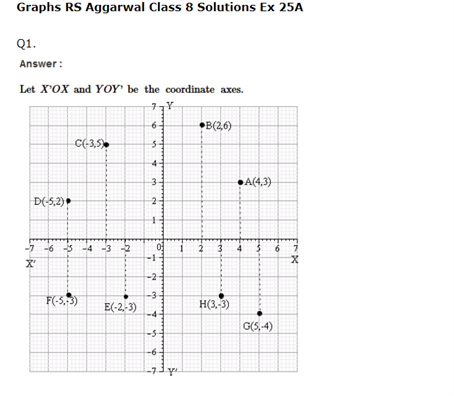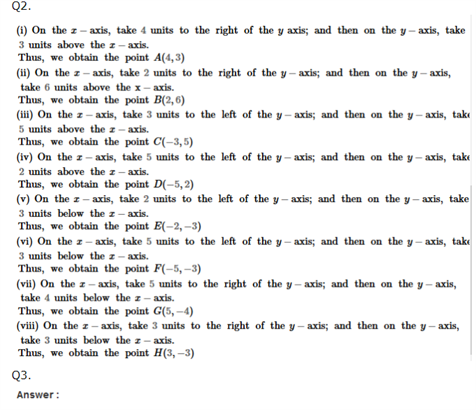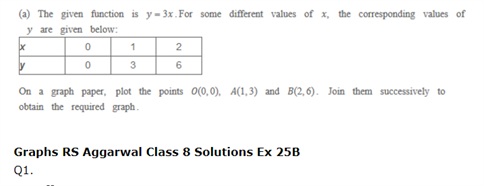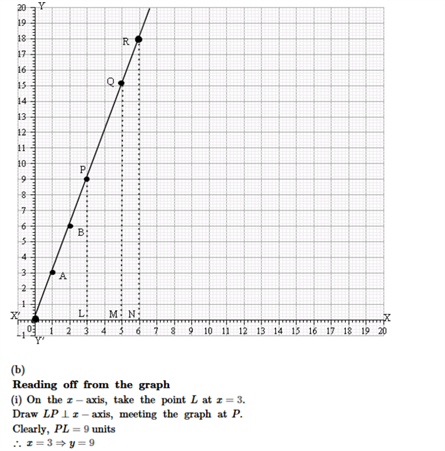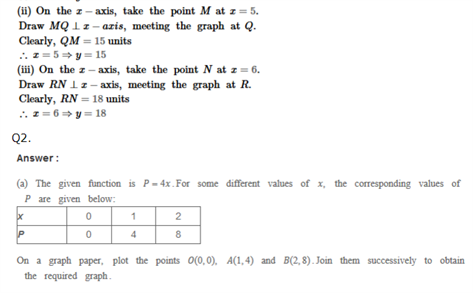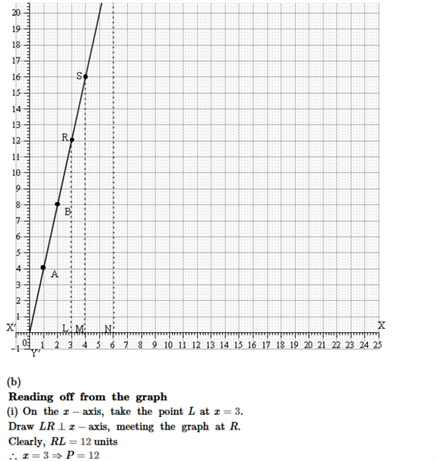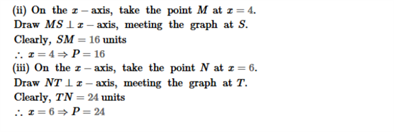Practise This QuestionThe capacity of a soda can be calculated using the formula.